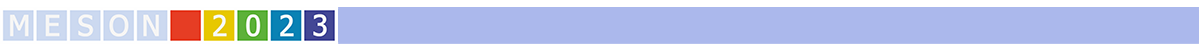#MESON2023

Jun 22 – 27, 2023
Auditorium Maximum
Europe/Warsaw timezone

## The $Y(4230)$ as a $D_1 D$ molecule

Jun 22, 2023, 5:55 PM
20m
Medium lecture hall (A) (Auditorium Maximum)

Parallel

### Speaker

Leon von Detten (Forschungszentrum Jülich)

### Description

We investigate the nature of the $Y(4230)$ in a simultaneous fit of the $DD^*\pi, J/\psi \pi \pi$ and $h_c \pi \pi$ crosssections and invariant mass spectra of the subsystems. We show to what extend a single $D_1 D$ bound state can explain the non-trivial asymmetric lineshape observed in these channels. At the same time we study if the $Z_c(3900)$ observed in the $J/\psi \pi$ invariant mass distribution can be understood as a $D^* D$ molecule or is a simple re-scattering effect.

### Primary authors

Christoph Hanhart (Forschungszentrum Jülich) Leon von Detten (Forschungszentrum Jülich)